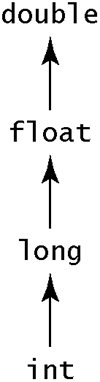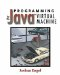# 10.8 Arithmetic Expressions

For arithmetic expressions in Java, the Java virtual machine instruction set has an instruction corresponding to the operator and the type of the arguments. Table 10.6 summarizes the choices. Although each binary operator requires two subexpressions, only one type is given in the table. That's because it's assumed that both subexpressions have the same type. If the expressions have different types, then one must be coerced to have the same type as the other.

#### 10.8.1 Numeric Coercions

Coercion is the process of converting a value of one type into a value of a different type. Although the two values are completely different to the JVM, they mean something similar to the user. The number 1.0 (the floating-point number 1) is completely different from the number 1 (the integer 1); arithmetic instructions that apply to one of them do not apply to the other. However, it is clear that 1.0 and 1 are corresponding values in the different domains of numbers.

For example, consider this Java expression:

` 1.0 + 1 `

According to The Java Language Specification, the result should be the floating-point number 2.0. The naïve transformation into bytecodes is this:

` fconst_1              ; Push 1.0 iconst_1              ; Push 1 fadd                  ; ERROR! Can't add a float to an int `

In order to make these two values have the same type, it is necessary to convert one of them to have the same type as the other. The primary goal is to preserve the magnitude of the number, and the secondary goal is to preserve the precision.

To accomplish this goal, the Java language specifies a hierarchy of numerical types:Because the floating-point types double and float are capable of representing both wide ranges of numbers and numbers with a fractional part, they are at the top of the hierarchy. The 64-bit types double and long appear above their 32-bit counterparts because the longer types are capable of holding more information. There are certain values of long that cannot be represented precisely with floats, especially very large numbers, but they can be represented with some loss of accuracy.

When an operator is applied to two numeric values, the one with the lower position in the hierarchy is converted so that it is "promoted" to the type of the other one. The conversion can be performed in a single instruction chosen according to Table 10.7.

Look again at the expression shown earlier:

` 1.0 + 1 `

To make this work, the int value must be promoted to match the float. From the table, the instruction to do this is i2f, which results in the code

` fconst_1              ; Push the float 1 iconst_1              ; Push the int 1 i2f                        ; Now the stack has two floats fadd                       ; This is now a valid instruction `

Suppose you have these declarations:

` long l; float f; int i; double d; `

Some other results are shown in Table 10.8. Note that the conversion depends only on the types of the operands. It is not necessary to look at which operand comes first. The operation being performed is also irrelevant.

The shorter int types char, byte, and short do not require any explicit conversion instructions to convert to ints. In the Java language, they are treated as distinct types; that is, char is not a subtype of int. In the JVM, however, they are treated as identical to ints. When an arithmetic operator is applied to these operators, they are automatically treated as full ints. Now suppose you have:

##### Table 10.7. Type conversion instructions
To From
int long float double
int - i2l i2f i2d
long l2i - l2f l2d
float f2i f2l - f2d
double d2i d2l d2f -

##### Table 10.9. Operations resulting in ints
Expression Conversion Result
c1+c2 None int
b1*b2 None int
s1/s2 None int
c1-s2 None int
b1/c2 None int

` byte b1, b2; short s1, s2; char c1, c2; `

Table 10.9 summarizes the expression types of various Java expressions. This makes sense from the point of view of a JVM bytecode programmer, since the JVM does not distinguish between int, byte, short, and char values. The JVM also treats booleans as identical to ints, but the Java language does not permit this:

` int i = 1;                // OK boolean b = true;         // OK int q = i+b           // ERROR! Can't add a boolean to an int int r = b;            // ERROR! Can't assign a boolean to an int `

You might expect that q would be assigned 2, since internally the value of b is represented by the int value 1, but this is not correct. The only operations that the Java language permits on boolean values are the boolean operators, which are discussed in section 10.11.

##### Table 10.8. Examples of numeric coercion
Expression Conversion Result
l+f Convert l to a float float
f+l Convert l to a float float
d/f Convert f to a double double
d*f Convert f to a double double
i*f Convert i to a float float
i*i None int
l*i Convert i to long long

#### 10.8.2 Casting Numeric Values

Only the upper half of Table 10.7 is used for promotions. The lower half contains demotions that convert doubles to floats or floats to ints and so on. These demotions are called narrowing.

The programmer may explicitly request a type coercion with a cast. For example,

` (int) 1.0 + 1 `

The result of this operation is the int 2, not the float 2.0. The value 1.0 is converted to an int, so when the + operation is performed it sees two int values. The code generated is:

` fconst_1              ; Push float 1.0 f2i                   ; Convert it to an int 1 iconst_1              ; Push int 1 iadd                  ; 1+1=2 `

The cast operation binds more tightly than the + operation. This expression is equivalent to

` ((int) 1.0) + 1 `

Parentheses can cause a different interpretation:

` (int) (1.0 + 1) `

In this case, there are two different conversions going on. An implicit conversion promotes 1 to a float, and then the two numbers are added together. Then the result of the addition is a float, which is then demoted to an int by the explicit cast. In Oolong, this is

` fconst_1               ; Push float 1 iconst_1               ; Push int 1 i2f                    ; Convert the int to a float fadd                   ; 1.0+1.0=2.0 f2i                    ; Convert 2.0 to 2 `

##### Table 10.10. Casting numeric types
Expression Result
(int) f Demote f to an int.
(double) f Promote f to a double.
(long) f Demote f to a long.
(float) l Promote l to a float.
(int) i No change
(double) i+f First i is converted to a float, then the final result is converted to a double.

The final result is the int 2. Although this simple case didn't show it, the order of operations is very important:

` (int) (1.5*2)        yields 3 `

but

` ((int) 1.5)*2        yields 2 `

In the first case, the 2 is converted to a float, and the multiplication operation is carried out on the float values before the result is converted to an int. In the second case, 1.5 is rounded to 1 when it's converted to an int, and 1*2 == 2.

Neither result is wrong; the programmer is given the option of choosing which result is desired when the program is written. This underscores the necessity of getting the order of operations correct.

Explicit casts may perform either promotions or demotions. If no change is called for, then no code is generated. Some results of casts, assuming the earlier definitions, are listed in Table 10.10. A few additional operations apply to conversions to short, char, or byte. Respectively, these are i2s, i2c, and i2b. They are generated only as a result of a specific cast:

` (byte) 4444         // Convert to 92 `

which compiles as

` ldc 4444          // Push the int 4444 i2b               // Convert to a byte `

The number is truncated to the appropriate number of bits (16 for i2s and i2c, 8 for i2b). For i2b and i2s, the resulting number is sign-extended, which means that the leftmost bit of the result after truncation is copied into the bits left of the truncation. This means that the char value is always positive, but the short and byte equivalents may be negative. Table 10.11 lists some examples of what happens when you cast an int to a smaller type.

#### 10.8.3 ~ Operator

The ~ operator is not represented in Table 10.6. The ~ operator takes an int or a long and inverts each bit. There is no instruction for this operator. Java compilers take note of the fact that, for a single bit x, computing ~x is equivalent to computing x + 1, where + is the exclusive-or operator. To invert all the bits in the number at once, the Java compiler uses the lxor or ixor instruction with the value consisting of 64 or 32 1's. In the two's complement notation used in the Java virtual machine, an integer consisting of all 1's is equal to 1. For example,

` ~x `

compiles to

` iload_1           ; Push x iconst_m1         ; Push -1 (1111...) ixor              ; Compute ~x `

If x is a long, ~x is

` lload_1         ; Push x ldc2_w -1L      ; Push -1 (1111...) lxor            ; Compute ~x `

##### Table 10.11. Converting between int types
Expression Result
(short) 65555 -1
(char) 65535 65535
(byte) 65535 -1
(short) 160 160
(char) 160 160
(byte) 160 -2Programming for the Javaв„ў Virtual Machine
ISBN: 0201309726
EAN: 2147483647
Year: 1998
Pages: 158
Authors: Joshua Engel

Similar book on Amazon